Integral Calculus -1

# Integral Calculus -1

Test Description

## 20 Questions MCQ Test Topic-wise Tests & Solved Examples for IIT JAM Mathematics | Integral Calculus -1

Integral Calculus -1 for IIT JAM 2023 is part of Topic-wise Tests & Solved Examples for IIT JAM Mathematics preparation. The Integral Calculus -1 questions and answers have been prepared according to the IIT JAM exam syllabus.The Integral Calculus -1 MCQs are made for IIT JAM 2023 Exam. Find important definitions, questions, notes, meanings, examples, exercises, MCQs and online tests for Integral Calculus -1 below.
Solutions of Integral Calculus -1 questions in English are available as part of our Topic-wise Tests & Solved Examples for IIT JAM Mathematics for IIT JAM & Integral Calculus -1 solutions in Hindi for Topic-wise Tests & Solved Examples for IIT JAM Mathematics course. Download more important topics, notes, lectures and mock test series for IIT JAM Exam by signing up for free. Attempt Integral Calculus -1 | 20 questions in 60 minutes | Mock test for IIT JAM preparation | Free important questions MCQ to study Topic-wise Tests & Solved Examples for IIT JAM Mathematics for IIT JAM Exam | Download free PDF with solutions
 1 Crore+ students have signed up on EduRev. Have you?
Integral Calculus -1 - Question 1

###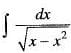is equal to

Detailed Solution for Integral Calculus -1 - Question 1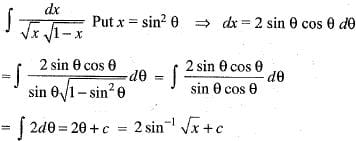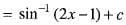Integral Calculus -1 - Question 2

###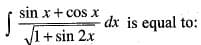Detailed Solution for Integral Calculus -1 - Question 2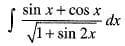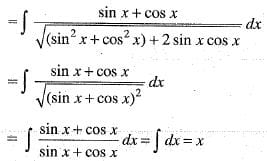Integral Calculus -1 - Question 3

###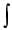x sec2 (x2 +1) tan (x2 +1) dx is equal to

Detailed Solution for Integral Calculus -1 - Question 3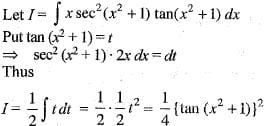Integral Calculus -1 - Question 4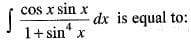Detailed Solution for Integral Calculus -1 - Question 4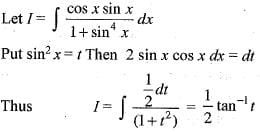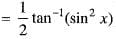Integral Calculus -1 - Question 5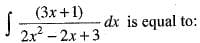Detailed Solution for Integral Calculus -1 - Question 5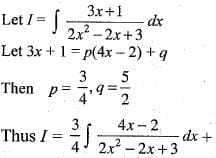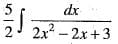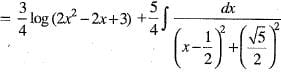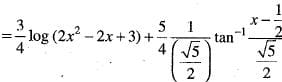Integral Calculus -1 - Question 6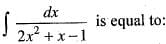Detailed Solution for Integral Calculus -1 - Question 6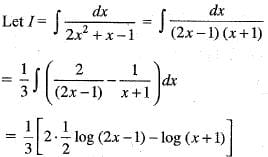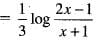Integral Calculus -1 - Question 7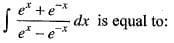Detailed Solution for Integral Calculus -1 - Question 7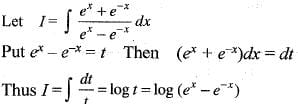Integral Calculus -1 - Question 8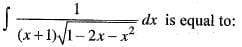Detailed Solution for Integral Calculus -1 - Question 8

Let us assume that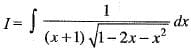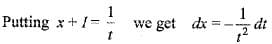Therefor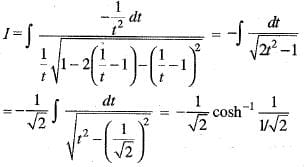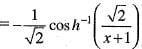Integral Calculus -1 - Question 9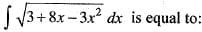Detailed Solution for Integral Calculus -1 - Question 9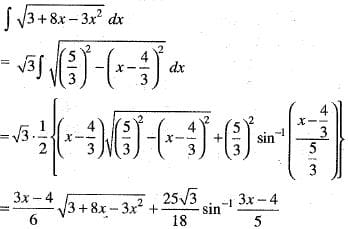Integral Calculus -1 - Question 10

The value of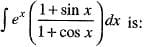Detailed Solution for Integral Calculus -1 - Question 10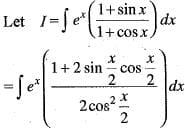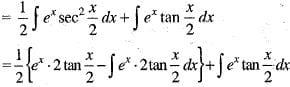Integral Calculus -1 - Question 11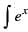sec x(1 + tan x) dx is equal to

Detailed Solution for Integral Calculus -1 - Question 11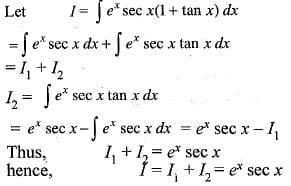Integral Calculus -1 - Question 12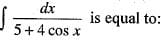Detailed Solution for Integral Calculus -1 - Question 12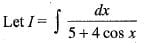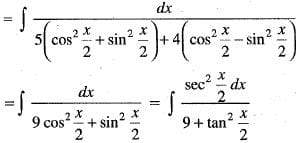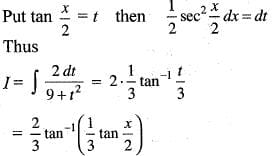Integral Calculus -1 - Question 13

As n —> ∞ the expression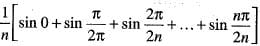is equal to

Detailed Solution for Integral Calculus -1 - Question 13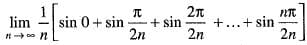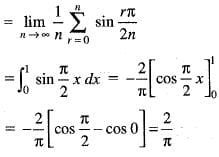Integral Calculus -1 - Question 14

The value of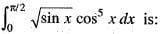Detailed Solution for Integral Calculus -1 - Question 14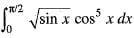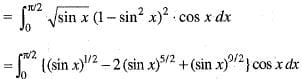Put sin x = t
=> cos x dx = dt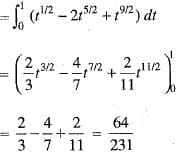Integral Calculus -1 - Question 15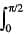log tan xdx is equal to:

Detailed Solution for Integral Calculus -1 - Question 15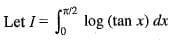... (i)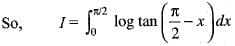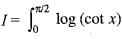... (ii)
Adding (i) and (ii), we get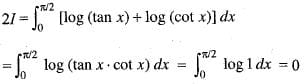Hence,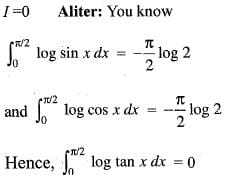Integral Calculus -1 - Question 16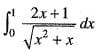id equal to

Detailed Solution for Integral Calculus -1 - Question 16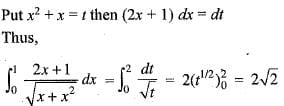Integral Calculus -1 - Question 17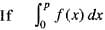= K, then the value of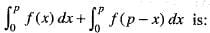Integral Calculus -1 - Question 18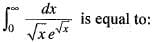Detailed Solution for Integral Calculus -1 - Question 18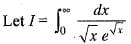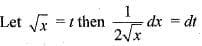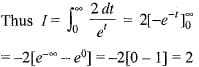Integral Calculus -1 - Question 19

Using the definition of integration as a process of summation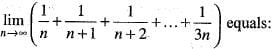Detailed Solution for Integral Calculus -1 - Question 19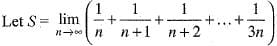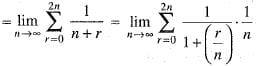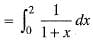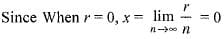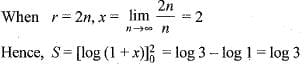Integral Calculus -1 - Question 20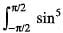x dx is equal to

## Topic-wise Tests & Solved Examples for IIT JAM Mathematics

27 docs|150 tests
 Use Code STAYHOME200 and get INR 200 additional OFF Use Coupon Code
Information about Integral Calculus -1 Page
In this test you can find the Exam questions for Integral Calculus -1 solved & explained in the simplest way possible. Besides giving Questions and answers for Integral Calculus -1, EduRev gives you an ample number of Online tests for practice

## Topic-wise Tests & Solved Examples for IIT JAM Mathematics

27 docs|150 tests